# Statistics review 8: Qualitative data – tests of association

## Abstract

This review introduces methods for investigating relationships between two qualitative (categorical) variables. The χ2 test of association is described, together with the modifications needed for small samples. The test for trend, in which at least one of the variables is ordinal, is also outlined. Risk measurement is discussed. The calculation of confidence intervals for proportions and differences between proportions are described. Situations in which samples are matched are considered.

## Introduction

In the previous statistics reviews most of the procedures discussed are appropriate for quantitative measurements. However, qualitative, or categorical, data are frequently collected in medical investigations. For example, variables assessed might include sex, blood group, classification of disease, or whether the patient survived. Categorical variables may also comprise grouped quantitative variables, for example age could be grouped into 'under 20 years', '20–50 years' and 'over 50 years'. Some categorical variables may be ordinal, that is the data arising can be ordered. Age group is an example of an ordinal categorical variable.

When using categorical variables in an investigation, the data can be summarized in the form of frequencies, or counts, of patients in each category. If we are interested in the relationship between two variables, then the frequencies can be presented in a two-way, or contingency, table. For example, Table 1 comprises the numbers of patients in a two-way classification according to site of central venous cannula and infectious complications. Interest here is in whether there is any relationship, or association, between the site of cannulation and the incidence of infectious complications. The question could also be phrased in terms of proportions, for example whether the proportions of patients in the three groups determined by site of central venous cannula differ according to type of infectious complication.

## χ2 test of association

In order to test whether there is an association between two categorical variables, we calculate the number of individuals we would get in each cell of the contingency table if the proportions in each category of one variable remained the same regardless of the categories of the other variable. These values are the frequencies we would expect under the null hypothesis that there is no association between the variables, and they are called the expected frequencies. For the data in Table 1, the proportions of patients in the sample with cannulae sited at the internal jugular, subclavian and femoral veins are 934/1706, 524/1706, 248/1706, respectively. There are 1305 patients with no infectious complications. So the frequency we would expect in the internal jugular site category is 1305 × (934/1706) = 714.5. Similarly for the subclavian and femoral sites we would expect frequencies of 1305 × (524/1706) = 400.8 and 1305 × (248/1706) = 189.7.

We repeat these calculations for the patients with infections at the exit site and with bacteraemia/septicaemia to obtain the following:

Exit site: 245 × (934/1706) = 134.1, 245 × (524/1706) = 75.3, 245 × 248/1706 = 35.6

Bacteraemia/septicaemia: 156 × (934/1706) = 85.4, 156 × (524/1706) = 47.9, 156 × (248/1706) = 22.7

We thus obtain a table of expected frequencies (Table 2). Note that 1305 × (934/1706) is the same as 934 × (1305/8766), and so equally we could have worded the argument in terms of proportions of patients in each of the infectious complications categories remaining constant for each central line site. In each case, the calculation is conditional on the sizes of the row and column totals and on the total sample size.

The test of association involves calculating the differences between the observed and expected frequencies. If the differences are large, then this suggests that there is an association between one variable and the other. The difference for each cell of the table is scaled according to the expected frequency in the cell. The calculated test statistic for a table with r rows and c columns is given by: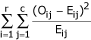where Oij is the observed frequency and Eij is the expectedfrequency in the cell in row i and column j. If the null hypothesis of no association is true, then the calculated test statistic approximately follows a χ2 distribution with (r - 1) × (c - 1) degrees of freedom (where r is the number of rows and c the number of columns). This approximation can be used to obtain a P value.

For the data in Table 1, the test statistic is:

1.134 + 2.380 + 1.314 + 6.279 + 21.531 + 2.052 + 2.484 + 14.069 + 0.020 = 51.26

Comparing this value with a χ2 distribution with (3 - 1) × (3 - 1) = 4 degrees of freedom, a P value of less than 0.001 is obtained either by using a statistical package or referring to a χ2 table (such as Table 3), in which 51.26 being greater than 18.47 leads to the conclusion that P < 0.001. Thus, there is a probability of less than 0.001 of obtaining frequencies like the ones observed if there were no association between site of central venous line and infectious complication. This suggests that there is an association between site of central venous line and infectious complication.

## Residuals

The χ2 test indicates whether there is an association between two categorical variables. However, unlike the correlation coefficient between two quantitative variables (see Statistics review 7 ), it does not in itself give an indication of the strength of the association. In order to describe the association more fully, it is necessary to identify the cells that have large differences between the observed and expected frequencies. These differences are referred to as residuals, and they can be standardized and adjusted to follow a Normal distribution with mean 0 and standard deviation 1 . The adjusted standardized residuals, dij, are given by: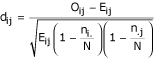Where ni. is the total frequency for row i, n.j is the total frequency for column j, and N is the overall total frequency. In the example, the adjusted standardized residual for those with cannulae sited at the internal jugular and no infectious complications is calculated as: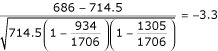Table 4 shows the adjusted standardized residuals for each cell. The larger the absolute value of the residual, the larger the difference between the observed and expected frequencies, and therefore the more significant the association between the two variables. Subclavian site/no infectious complication has the largest residual, being 6.2. Because it is positive there are more individuals than expected with no infectious complications where the subclavian central line site was used. As these residuals follow a Normal distribution with mean 0 and standard deviation 1, all absolute values over 2 are significant (see Statistics review 2 ). The association between femoral site/no infectious complication is also significant, but because the residual is negative there are fewer individuals than expected in this cell. When the subclavian central line site was used infectious complications appear to be less likely than when the other two sites were used.

## Two by two tables

The use of the χ2 distribution in tests of association is an approximation that depends on the expected frequencies being reasonably large. When the relationship between two categorical variables, each with only two categories, is being investigated, variations on the χ2test of association are often calculated as well as, or instead of, the usual test in order to improve the approximation. Table 5 comprises data on patients with acute myocardial infarction who took part in a trial of intravenous nitrate (see Statistics review 3 ). A total of 50 patients were randomly allocated to the treatment group and 45 to the control group. The table shows the numbers of patients who died and survived in each group. The χ2 test gives a test statistic of 3.209 with 1 degree of freedom and a P value of 0.073. This suggests there is not enough evidence to indicate an association between treatment and survival.

### Fisher's exact test

The exact P value for a two by two table can be calculated by considering all the tables with the same row and column totals as the original but which are as or more extreme in their departure from the null hypothesis. In the case of Table 5, we consider all the tables in which three or fewer patients receiving the treatment died, given in Table 6(i)–(iv). The exact probabilities of obtaining each of these tables under the null hypothesis of no association or independence between treatment and survival are obtained as follows.

To calculate the probability of obtaining a particular table, we consider the total number of possible tables with the given marginal totals, and the number of ways we could have obtained the particular cell frequencies in the table in question. The number of ways the row totals of 11 and 84 could have been obtained given 95 patients altogether is denoted by 95C11 and is equal to 95!/11!84!, where 95! ('95 factorial') is the product of 95 and all the integers lower than itself down to 1. Similarly the number of ways the column totals of 50 and 45 could have been obtained is given by 95C50 = 95!/50!45!. Assuming independence, the total number of possible tables with the given marginal totals is: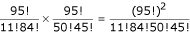The number of ways Table 5 (Table 6[i]) could have been obtained is given by considering the number of ways each cell frequency could have arisen. There are 95C3 ways of obtaining the three patients in the first cell. The eight patients in the next cell can be obtained in 92C8 ways from the 95 - 3 = 92 remaining patients. The remaining cells can be obtained in 84C47 and 37C37 (= 1) ways. Therefore, the number of ways of obtaining Table 6(i) under the null hypothesis is: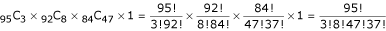Therefore the probability of obtaining 6(i) is:

Therefore the total probability of obtaining the four tables given in Table 6 is: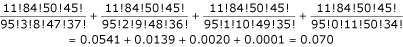This probability is usually doubled to give a two-sided P value of 0.140. There is quite a large discrepancy in this case between the χ2 test and Fisher's exact test.

### Yates' continuity correction

In using the χ2 distribution in the test of association, a continuous probability distribution is being used to approximate discrete probabilities. A correction, attributable to Yates, can be applied to the frequencies to make the test closer to the exact test. To apply Yates' correction for continuity we increase the smallest frequency in the table by 0.5 and adjust the other frequencies accordingly to keep the row and column totals the same. Applying this correction to the data given in Table 5 gives Table 7.

The χ2 test using these adjusted figures gives a test statistic of 2.162 with a P value of 0.141, which is close to the P value for Fisher's exact test.

For large samples the three tests – χ2, Fisher's and Yates' – give very similar results, but for smaller samples Fisher's test and Yates' correction give more conservative results than the χ2 test; that is the P values are larger, and we are less likely to conclude that there is an association between the variables. There is some controversy about which method is preferable for smaller samples, but Bland  recommends the use of Fisher's or Yates' test for a more cautious approach.

## Test for trend

Table 8 comprises the numbers of patients in a two-way classification according to AVPU classification (voice and pain responsive categories combined) and subsequent survival or death of 1306 patients attending an accident and emergency unit. (AVPU is a system for assessing level of consciousness: A = alert, V = voice responsiveness, P = pain responsive and U = unresponsive.) The χ2 test of association gives a test statistic of 19.38 with 2 degrees of freedom and a P value of less than 0.001, suggesting that there is an association between survival and AVPU classification.

Because the categories of AVPU have a natural ordering, it is appropriate to ask whether there is a trend in the proportion dying over the levels of AVPU. This can be tested by carrying out similar calculations to those used in regression for testing the gradient of a line (see Statistics review 7 ). Suppose the variable 'survival' is regarded as the y variable taking two values, 1 and 2 (survived and died), and AVPU as the x variable taking three values, 1, 2 and 3. We then have six pairs of x, y values, each occurring the number of times equal to the frequency in the table; for example, we have 1110 occurrences of the point (1,1).

Following the lines of the test of the gradient in regression, with some fairly minor modifications and using large sample approximations, we obtain a χ2 statistic with 1 degree of freedom given by :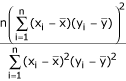For the data in Table 8, we obtain a test statistic of 19.33 with 1 degree of freedom and a P value of less than 0.001. Therefore, the trend is highly significant. The difference between the χ2 test statistic for trend and the χ2 test statistic in the original test is 19.38 - 19.33 = 0.05 with 2 - 1 = 1 degree of freedom, which provides a test of the departure from the trend. This departure is very insignificant and suggests that the association between survival and AVPU classification can be explained almost entirely by the trend.

Some computer packages give the trend test, or a variation. The trend test described above is sometimes called the Cochran–Armitage test, and a common variation is the Mantel–Haentzel trend test.

## Measurement of risk

Another application of a two by two contingency table is to examine the association between a disease and a possible risk factor. The risk for developing the disease if exposed to the risk factor can be calculated from the table. A basic measurement of risk is the probability of an individual developing a disease if they have been exposed to a risk factor (i.e. the relative frequency or proportion of those exposed to the risk factor that develop the disease). For example, in the study into early goal-directed therapy in the treatment of severe sepsis and septic shock conducted by Rivers and coworkers , one of the outcomes measured was in-hospital mortality. Of the 263 patients who were randomly allocated either to early goal-directed therapy or to standard therapy, 236 completed the therapy period with the outcomes shown in Table 9.

From the table it can be seen that the proportion of patients receiving early goal-directed therapy who died is 38/117 = 32.5%, and so this is the risk for death with early goal-directed therapy. The risk for death on the standard therapy is 59/119 = 49.6%.

Another measurement of the association between a disease and possible risk factor is the odds. This is the ratio of those exposed to the risk factor who develop the disease compared with those exposed to the risk factor who do not develop the disease. This is best illustrated by a simple example. If a bag contains 8 red balls and 2 green balls, then the probability (risk) of drawing a red ball is 8/10 whereas the odds of drawing a red ball is 8/2. As can be seen, the measurement of odds, unlike risk, is not confined to the range 0–1. In the study conducted by Rivers and coworkers  the odds of death with early goal-directed therapy is 38/79 = 0.48, and on the standard therapy it is 59/60 = 0.98.

### Confidence interval for a proportion

As the measurement of risk is simply a proportion, the confidence interval for the population measurement of risk can be calculated as for any proportion. If the number of individuals in a random sample of size n who experience a particular outcome is r, then r/n is the sample proportion, p. For large samples the distribution of p can be considered to be approximately Normal, with a standard error of :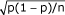The 95% confidence interval for the true population proportion, p, is given by p - 1.96 × standard error to p + 1.96 × standard error, which is: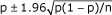where p is the sample proportion and n is the sample size. The sample proportion is the risk and the sample size is the total number exposed to the risk factor.

For the study conducted by Rivers and coworkers  the 95% confidence interval for the risk for death on early goal-directed therapy is 0.325 ± 1.96(0.325 [1-0.325]/117)0.5 or (24.0%, 41.0%), and on the standard therapy it is (40.6%, 58.6%). The interpretation of a confidence interval is described in (see Statistics review 2 ) and indicates that, for those on early goal-directed therapy, the true population risk for death is likely to be between 24.0% and 41.0%, and that for the standard therapy between 40.6% and 58.6%.

## Comparing risks

To assess the importance of the risk factor, it is necessary to compare the risk for developing a disease in the exposed group with the risk in the nonexposed group. In the study by Rivers and coworkers  the risk for death on the early goal-directed therapy is 32.5%, whereas on the standard therapy it is 49.6%. A comparison between the two risks can be made by examining either their ratio or the difference between them.

### Risk ratio

The risk ratio measures the increased risk for developing a disease when having been exposed to a risk factor compared with not having been exposed to the risk factor. It is given by RR = risk for the exposed/risk for the unexposed, and it is often referred to as the relative risk. The interpretation of a relative risk is described in Statistics review 6 . For the Rivers study the relative risk = 0.325/0.496 = 0.66, which indicates that a patient on the early goal-directed therapy is 34% less likely to die than a patient on the standard therapy.

The calculation of the 95% confidence interval for the relative risk  will be covered in a future review, but it can usefully be interpreted here. For the Rivers study the 95% confidence interval for the population relative risk is 0.48 to 0.90. Because the interval does not contain 1.0 and the upper end is below, it indicates that patients on the early goal-directed therapy have a significantly decreased risk for dying as compared with those on the standard therapy.

### Odds ratio

When quantifying the risk for developing a disease, the ratio of the odds can also be used as a measurement of comparison between those exposed and not exposed to a risk factor. It is given by OR = odds for the exposed/odds for the unexposed, and is referred to as the odds ratio. The interpretation of odds ratio is described in Statistics review 3 . For the Rivers study the odds ratio = 0.48/0.98 = 0.49, again indicating that those on the early goal-directed therapy have a reduced risk for dying as compared with those on the standard therapy. This will be covered fully in a future review.

The calculation of the 95% confidence interval for the odds ratio  will also be covered in a future review but, as with relative risk, it can usefully be interpreted here. For the Rivers example the 95% confidence interval for the odds ratio is 0.29 to 0.83. This can be interpreted in the same way as the 95% confidence interval for the relative risk, indicating that those receiving early goal-directed therapy have a reduced risk for dying.

### Difference between two proportions

#### Confidence interval

For the Rivers study, instead of examining the ratio of the risks (the relative risk) we can obtain a confidence interval and carry out a significance test of the difference between the risks. The proportion of those on early goal-directed therapy who died is p1 = 38/117 = 0.325 and the proportion of those on standard therapy who died is p2 = 59/119 = 0.496. A confidence interval for the difference between the true population proportions is given by:

(p1 - p2) - 1.96 × se(p1 - p2) to (p1 - p2) + 1.96 × se(p1 - p2)

Where se(p1 - p2) is the standard error of p1 - p2 and is calculated as: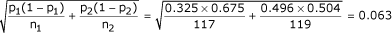Thus, the required confidence interval is -0.171 - 1.96 × 0.063 to -0.171 + 1.96 × 0.063; that is -0.295 to -0.047. Therefore, the difference between the true proportions is likely to be between -0.295 and -0.047, and the risk for those on early goal-directed therapy is less than the risk for those on standard therapy.

#### Hypothesis test

We can also carry out a hypothesis test of the null hypothesis that the difference between the proportions is 0. This follows similar lines to the calculation of the confidence interval, but under the null hypothesis the standard error of the difference in proportions is given by: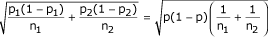where p is a pooled estimate of the proportion obtained from both samples :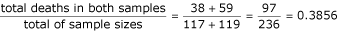So:The test statistic is then: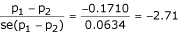Comparing this value with a standard Normal distribution gives p = 0.007, again suggesting that there is a difference between the two population proportions. In fact, the test described is equivalent to the χ2test of association on the two by two table. The χ2 test gives a test statistic of 7.31, which is equal to (-2.71)2 and has the same P value of 0.007. Again, this suggests that there is a difference between the risks for those receiving early goal-directed therapy and those receiving standard therapy.

## Matched samples

Matched pair designs, as discussed in Statistics review 5 , can also be used when the outcome is categorical. For example, when comparing two tests to determine a particular condition, the same individuals can be used for each test.

### McNemar's test

In this situation, because the χ2 test does not take pairing into consideration, a more appropriate test, attributed to McNemar, can be used when comparing these correlated proportions.

For example, in the comparison of two diagnostic tests used in the determination of Helicobacter pylori, the breath test and the Oxoid test, both tests were carried out in 84 patients and the presence or absence of H. pylori was recorded for each patient. The results are shown in Table 10, which indicates that there were 72 concordant pairs (in which the tests agree) and 12 discordant pairs (in which the tests disagree). The null hypothesis for this test is that there is no difference in the proportions showing positive by each test. If this were true then the frequencies for the two categories of discordant pairs should be equal . The test involves calculating the difference between the number of discordant pairs in each category and scaling this difference by the total number of discordant pairs. The test statistic is given by: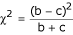Where b and c are the frequencies in the two categories of discordant pairs (as shown in Table 10). The calculated test statistic is compared with a χ2 distribution with 1 degree of freedom to obtain a P value. For the example b = 8 and c = 4, therefore the test statistic is calculated as 1.33. Comparing this with a χ2 distribution gives a P value greater than 0.10, indicating no significant difference in the proportion of positive determinations of H. pylori using the breath and the Oxoid tests.

The test can also be carried out with a continuity correction attributed to Yates , in a similar way to that described above for the χ2test of association. The test statistic is then given by: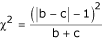and again is compared with a χ2 distribution with 1 degree of freedom. For the example, the calculated test statistic including the continuity correct is 0.75, giving a P value greater than 0.25.

As with nonpaired proportions a confidence interval for the difference can be calculated. For large samples the difference between the paired proportions can be approximated to a Normal distribution. The difference between the proportions can be calculated from the discordant pairs , so the difference is given by (b - c)/n, where n is the total number of pairs, and the standard error of the difference by (b + c)0.5/n.

For the example where b = 8, c = 4 and n = 84, the difference is calculated as 0.048 and the standard error as 0.041. The approximate 95% confidence interval is therefore 0.048 ± 1.96 × 0.041 giving -0.033 to 0.129. As this spans 0, it again indicates that there is no difference in the proportion of positive determinations of H. pylori using the breath and the Oxoid tests.

## Limitations

For a χ2 test of association, a recommendation on sample size that is commonly used and attributed to Cochran  is that no cell in the table should have an expected frequency of less than one, and no more than 20% of the cells should have an expected frequency of less than five. If the expected frequencies are too small then it may be possible to combine categories where it makes sense to do so.

For two by two tables, Yates' correction or Fisher's exact test can be used when the samples are small. Fisher's exact test can also be used for larger tables but the computation can become impossibly lengthy.

In the trend test the individual cell sizes are not important but the overall sample size should be at least 30.

The analyses of proportions and risks described above assume large samples with similar requirement to the χ2 test of association .

The sample size requirement often specified for McNemar's test and confidence interval is that the number of discordant pairs should be at least 10 .

## Conclusion

The χ2 test of association and other related tests can be used in the analysis of the relationship between categorical variables. Care needs to be taken to ensure that the sample size is adequate.

## Box

Previous articles have covered 'presenting and summarizing data', 'samples and populations', 'hypothesestesting and P values', 'sample size calculations', 'comparison of means', 'nonparametric means' and 'correlation and regression'.

Future topics to be covered include:

Chi-squared and Fishers exact tests

Analysis of variance

Further non-parametric tests: Kruskal–Wallis and Friedman

Measures of disease: PR/OR

Survival data: Kaplan–Meier curves and log rank tests

ROC curves

Multiple logistic regression.

If there is a medical statistics topic you would like explained, contact us at editorial@ccforum.com.

## Abbreviations

AVPU:

A = alert, V = voice responsiveness, P = pain responsive and U = unresponsive

## References

1. Bewick V, Cheek L, Ball J: Statistics review 7: Correlation and regression. Crit Care 2003, 7: 451-459. 10.1186/cc2401

2. Everitt BS: The Analysis of Contingency Tables 2 Edition London, UK: Chapman & Hall 1992.

3. Whitley E, Ball J: Statistics review 2: samples and populations. Crit Care 2002, 6: 143-148. 10.1186/cc1473

4. Whitley E, Ball J: Statistics review 3: hypothesis testing and P values. Crit Care 2002, 6: 222-225. 10.1186/cc1493

5. Bland M: An Introduction to Medical Statistics 3 Edition Oxford, UK: Oxford University Press 2001.

6. Rivers E, Nguyen B, Havstad S, Ressler J, Muzzin A, Knoblich B, Peterson E, Tomlanovich M, Early Goal-Directed Therapy Collaborative Group: Early goal-directed therapy in the treatment of severe sepsis and septic shock. N Engl J Med 2001, 345: 1368-1377. 10.1056/NEJMoa010307

7. Whitley E, Ball J: Statistics review 6: Nonparametric methods. Crit Care 2002, 6: 509-513. 10.1186/cc1820

8. Kirkwood BR, Sterne JAC: Essential Medical Statistics 2 Edition Oxford, UK: Blackwell Science Ltd 2003.

9. Whitley E, Ball J: Statistics review 5: Comparison of means. Crit Care 2002, 6: 424-428. 10.1186/cc1548

## Author information

Authors

### Corresponding author

Correspondence to Viv Bewick.

None declared.

## Rights and permissions

Reprints and Permissions

Bewick, V., Cheek, L. & Ball, J. Statistics review 8: Qualitative data – tests of association. Crit Care 8, 46 (2003). https://doi.org/10.1186/cc2428

• Published:

• DOI: https://doi.org/10.1186/cc2428

### Keywords

• χ2 test of association
• Fisher's exact test
• McNemar's test
• odds ratio
• risk ratio
• Yates' correction